The Inscribed Shapes ClipArt gallery provides 86 examples of geometric spapes enclosed within another shape with every vertex of the enclosed figure touching the outer figure.### Square Inscribed in a 12-Point Star

Illustration of a square inscribed in a closed concave geometric figure with 24 sides in the shape of…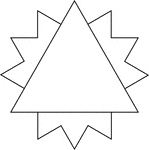### Triangle Inscribed In A 12-Point Star

Illustration of an equilateral triangle inscribed in a closed concave geometric figure with 24 sides…### Circle Triangle Tangents

Six equal circles tangent to each other and to the sides of the triangle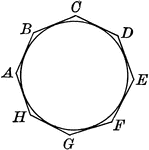### Circle with Circumscribed Polygon

Illustrations of a circle with a circumscribed polygon. Or, a circle inscribed in an octagon.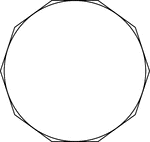### Decagon Circumscribed About A Circle

Illustration of a decagon circumscribed about a circle. This can also be described as a circle inscribed…### Decagon Inscribed In A Circle

Illustration of a decagon inscribed in a circle. This can also be described as a circle circumscribed…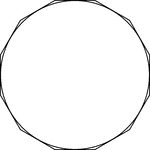### Dodecagon Circumscribed About A Circle

Illustration of a dodecagon circumscribed about a circle. This can also be described as a circle inscribed…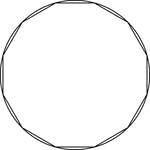### Dodecagon Inscribed In A Circle

Illustration of a dodecagon inscribed in a circle. This can also be described as a circle circumscribed…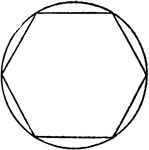### Hexagon Inscribed in Circle

Illustration of hexagon inscribed in circle. Or, circle circumscribed about hexagon.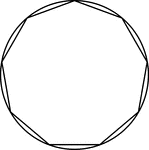### Nonagon Inscribed in a Circle

Illustration of a regular nonagon inscribed in a circle. This could also be described as a circle circumscribed…### Pentagon Inscribed in Circle

Illustration of pentagon inscribed in circle. Or, circle circumscribed about pentagon.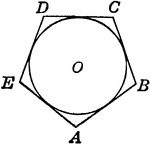Illustration of polygon circumscribed about circle. Or, circle inscribed in the polygon.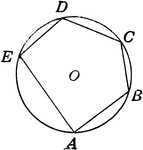### Polygon Inscribed in Circle

Illustration of polygon inscribed in circle. Or, circle circumscribed about the polygon.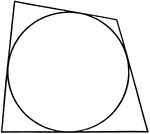Illustration of a quadrilateral circumscribed about a circle. This could also be described as a circle…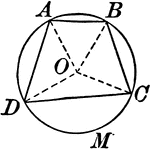Illustration showing a circle with a quadrilateral inscribed.### Quadrilateral Inscribed In A Circle

An illustration showing a quadrilateral inscribed in a circle that is tangent to a line.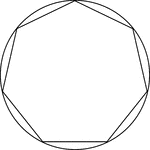### Regular Heptagon/Septagon Inscribed In A Circle

Illustration of a regular heptagon/septagon inscribed in a circle. This can also be described as a circle…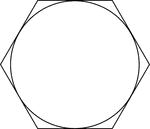### Regular Hexagon Circumscribed About A Circle

Illustration of a regular hexagon circumscribed about a circle. This can also be described as a circle…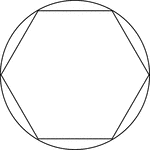### Regular Hexagon Inscribed In A Circle

Illustration of a regular hexagon inscribed in a circle. This can also be described as a circle circumscribed…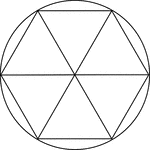### Regular Hexagon Inscribed In A Circle

Illustration of a regular hexagon inscribed in a circle. This can also be described as a circle circumscribed…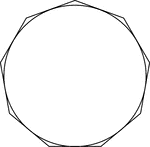### Regular Nonagon Circumscribed About A Circle

Illustration of a regular nonagon circumscribed about a circle. This can also be described as a circle…### Regular Nonagon Inscribed In A Circle

Illustration of a regular nonagon inscribed in a circle. This can also be described as a circle circumscribed…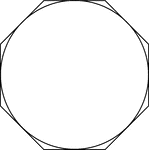### Regular Octagon Circumscribed About A Circle

Illustration of a regular octagon circumscribed about a circle. This can also be described as a circle…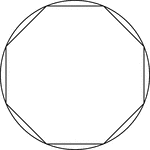### Regular Octagon Inscribed In A Circle

Illustration of a regular octagon inscribed in a circle. This can also be described as a circle circumscribed…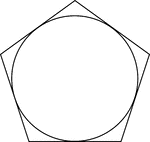### Regular Pentagon Circumscribed About A Circle

Illustration of a regular pentagon circumscribed about a circle. This can also be described as a circle…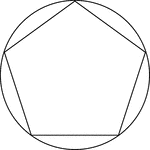### Regular Pentagon Inscribed In A Circle

Illustration of a regular pentagon inscribed in a circle. This can also be described as a circle circumscribed…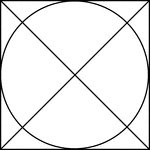### Square Circumscribed About A Circle

Illustration of a square, with diagonals drawn, circumscribed about a circle. This can also be described…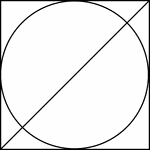### Square Circumscribed About A Circle

Illustration of a square, with 1 diagonals drawn, circumscribed about a circle. This can also be described…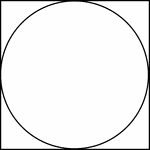### Square Circumscribed About A Circle

Illustration of a square circumscribed about a circle. This can also be described as a circle inscribed…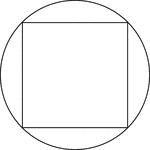### Square Inscribed In A Circle

Illustration of a square inscribed in a circle. This can also be described as a circle circumscribed…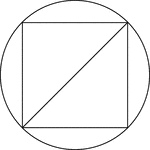### Square Inscribed In A Circle

Illustration of a square inscribed in a circle. This can also be described as a circle circumscribed…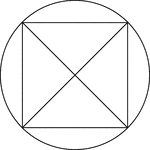### Square Inscribed In A Circle

Illustration of a square, with diagonals drawn, inscribed in a circle. This can also be described as…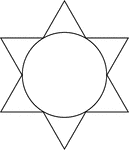### Star Circumscribed About A Circle

Illustration of a 6-point star (convex dodecagon) circumscribed about a circle. This can also be described…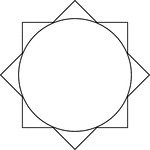### Star Circumscribed About A Circle

Illustration of an 8-point star (convex polygon) circumscribed about a circle. This can also be described…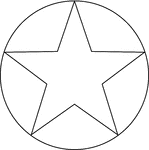### Star Inscribed In A Circle

Illustration of a 5-point star inscribed in a circle. This can also be described as a circle circumscribed…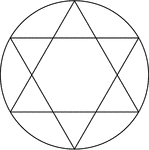### Star Inscribed In A Circle

Illustration of a 6-point star created by two equilateral triangles (often described as the Star of…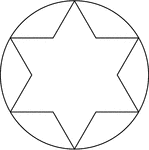### Star Inscribed In A Circle

Illustration of a 6-point star (convex dodecagon) inscribed in a circle. This can also be described…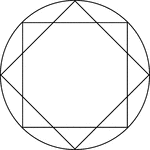### Star Inscribed In A Circle

Illustration of an 8-point star, created by two squares at 45° rotations, inscribed in a circle.…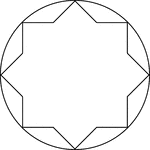### Star Inscribed In A Circle

Illustration of an 8-point star, or convex polygon, inscribed in a circle. This can also be described…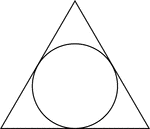### Triangle Circumscribed About A Circle

Illustration of an equilateral triangle circumscribed about a circle. This can also be described as…### Triangle Inscribed in Circle

Illustration of triangle inscribed in circle. Or, circle circumscribed about triangle.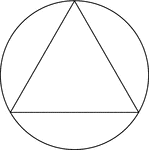### Triangle Inscribed In A Circle

Illustration of an equilateral triangle inscribed in a circle. This can also be described as a circle…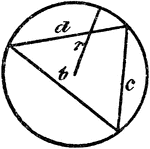### Triangle Inscribed In A Circle

An illustration showing a triangle with sides b, c, and d inscribed in a circle with radius r.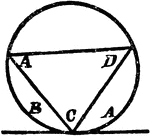### Triangle Inscribed In A Circle

An illustration showing a triangle with angles A, C, and D inscribed in a circle which is tangent to…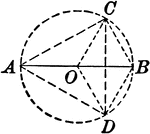### Circle With Inscribed Triangle and Radii

Illustration showing a circle with an inscribed triangle and radii.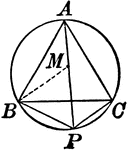### Circle With Inscribed Equilateral Triangle

Illustration showing an equilateral triangle inscribed in a circle.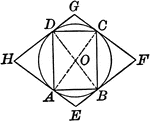### Circle With Inscribed and Circumscribed Quadrilaterals

Illustration showing a circle with an inscribed and circumscribed quadrilateral.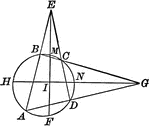### Circle With Inscribed Quadrilateral and Triangles Formed

Illustration showing a circle with an inscribed quadrilateral and triangles formed by extended chords.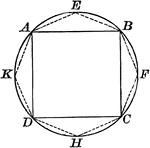### Circle With Polygon and Square Inscribed

Illustration of a circle with a polygon (octagon) and square inscribed.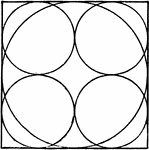### Circles Inside of a Square

Illustration of large circle and square with 4 smaller circles inside.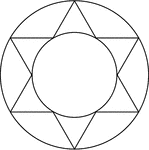### Star Inscribed And Circumscribed About Circles

Illustration of a 6-point star (convex dodecagon) inscribed in a large circle and circumscribed about…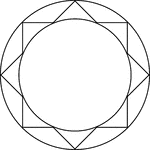### Star Inscribed And Circumscribed About Circles

Illustration of an 8-point star (convex polygon) inscribed in a large circle and circumscribed about…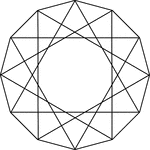### 12 Triangles Inscribed In A Dodecagon

Illustration of 12 equilateral triangles inscribed in an equilateral dodecagon. Each vertex of the dodecagon…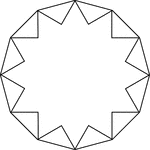### 12-Point Star Inscribed In A Dodecagon

Illustration of a 12-point star (24-sided polygon) inscribed in a regular dodecagon. This can also be…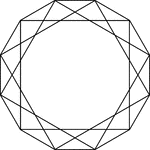### 3 Square Inscribed In A Dodecagon

Illustration of 3 squares inscribed in an regular dodecagon. Each vertex of the dodecagon is also a…### Hexagon And Triangle Inscribed In A Dodecagon

Illustration of a regular hexagon and an equilateral triangle inscribed in a regular dodecagon. This…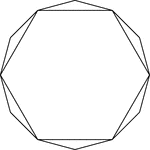### Hexagon Inscribed In A Dodecagon

Illustration of a regular hexagon inscribed in a regular dodecagon. This could also be described as…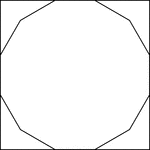### Square Circumscribed About A Dodecagon

Illustration of a square circumscribed about a regular dodecagon. This could also be described as a…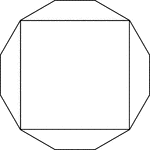### Square Inscribed In A Dodecagon

Illustration of a square inscribed in an regular dodecagon. This could also be described as a regular…